Our World Statistics Day conversations have been a great reminder of how much statistics can inform our lives. Do you have an example of how statistics has made a difference in your life? Share your story with the Community!
Choose Language Hide Translation Bar
Highlighted

## Graph Builder: hide X categories with no data

Hello everyone,

I have a table with a grouping column and several response variables.

``````New Table( "Test",
New Column( "GROUP",
Character,
"Nominal",
Set Values( {"a", "b", "c", "d", "e", "f", "g"} )
),
New Column( "Y",
Numeric,
"Continuous",
Format( "Best", 12 ),
Set Values( [7, ., 6, ., 4, ., 2] )
),
New Column( "Y 2",
Numeric,
"Continuous",
Format( "Best", 12 ),
Set Values( [7, 6, 2, 3, ., ., .] ),
Set Display Width( 49 )
)
)``````

I would like to create a bar chart with a column switcher.

``````Graph Builder(
Size( 527, 448 ),
Show Control Panel( 0 ),
Variables(
X( :GROUP, Order By( :Y, Ascending, Order Statistic( "Mean" ) ) ),
Y( :Y )
),
Elements( Bar( X, Y, Legend( 4 ) ) ),
Column Switcher( :Y, {:Y, :Y 2} )
)``````

My problem is that I do not want to display levels with no data. In other words, I do not want to see levels "b", "d" and "f" for Y and levels "e", "f", "g" for Y2.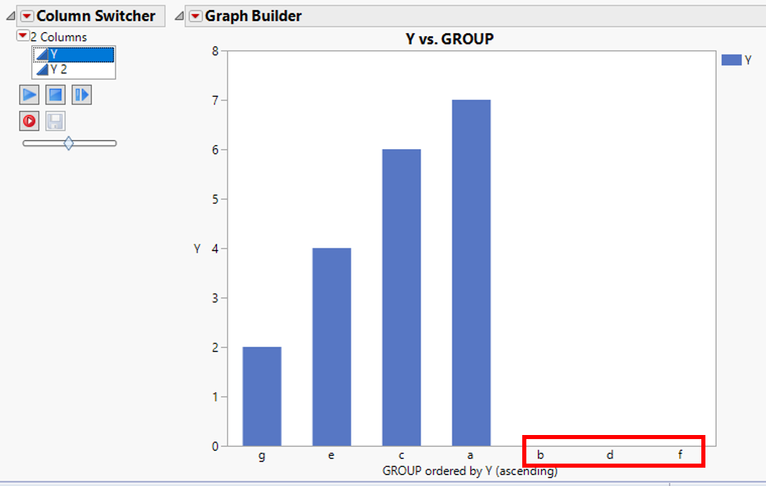I tried to use a "where" clause but unfortunately it is not taken into acount by the Column Switcher.

Do you have any idea of how we could do that?

1 ACCEPTED SOLUTION

Accepted Solutions
Highlighted

## Re: Graph Builder: hide X categories with no data

Hi again @anne_sa ,

Sorry for the delay, I actually caught the flu for a few days and got behind a little.

I have attached two files.  The first jsl file (Example Script for Changing row states.jsl) is the driver file and the path in the second line is the full path of the location that you place the second file (GraphBuilderJSLAPI.jsl).

``````//Include script to modify GraphBuilder with some special functions defined
include(ConvertFilePath("/Users/gemann/Projects/Prototypes/JSLClasses/GraphBuilderJSLAPI.jsl", absolute, windows));

//Create a sample table
dt = New Table( "Test",
New Column( "GROUP",
Character,
"Nominal",
Set Values( {"a", "b", "c", "d", "e", "f", "g"} )
),
New Column( "Y",
Numeric,
"Continuous",
Format( "Best", 12 ),
Set Values( [7, ., 6, ., 4, ., 2] )
),
New Column( "Y 2",
Numeric,
"Continuous",
Format( "Best", 12 ),
Set Values( [7, 6, 2, 3, ., ., .] ),
Set Display Width( 49 )
)
);

//Add a new window and build a listbox beside a graphbuilder
New Window( "CustomColumnSwitcher Window",
//Start by getting all of the columns in the current datatable
CNames = dt << Get Column Names( Numeric );
Show( CNames );

//User a horizontal layout
HListBox(
//Use a ListBox as the new Column Switcher
lb = List Box(
CNames,
maxSelected(1),
//The next lines specify the script that gets run when an item in listbox is selected
dt << clearrowstates();
newvarname = lb<<getselected();
ReplaceGBXVariable(gb,"GROUP",newvarname);
ReplaceGBYVariable(gb,newvarname);
rs = dt << select where( IsMissing( Column(newvarname)[] ) );
dt << exclude();
),
//Build the original GraphBuilder
gb = Graph Builder(
Size( 527, 448 ),
Show Control Panel( 0 ),
Variables(
X( :GROUP, Order By( :Y, Ascending, Order Statistic( "Mean" ) ) ),
Y( :Y )
),
Elements( Bar( X, Y, Legend( 4 ) ) )
);

// This line is added for the special case of filtering values that are missing from the graph
rs = currentdatatable() << select where(IsMissing(:Y));
dt << exclude();
);
);
``````

Good luck and let me know if you have any issues.  Of course these files are not fully tested!!!  :)

15 REPLIES 15
Highlighted

## Re: Graph Builder: hide X categories with no data

Hi Anne,

Your idea that you need a "where" clause or rather a filter of some sort is correct.  Try adding the following line to your graphbuilder script:

``````Local Data Filter( Add Filter( columns( :Y ), Where( :Y >= 2 & :Y <= 7 ) ) )
``````

I am hoping that a Local Data Filter is okay?

``````Graph Builder(
Size( 527, 448 ),
Show Control Panel( 0 ),
Variables(
X( :GROUP, Order By( :Y, Ascending, Order Statistic( "Mean" ) ) ),
Y( :Y )
),
Elements( Bar( X, Y, Legend( 4 ) ) ),
Column Switcher( :Y, {:Y, :Y 2} ),
Local Data Filter( Add Filter( columns( :Y ), Where( :Y >= 2 & :Y <= 7 ) ) )

);``````

Highlighted

## Re: Graph Builder: hide X categories with no data

Unfortunately is it not exactly what I would like to get because when I select a new column with the column switcher, the local data filter is still based on Y.

Of course it is possible to manually change the local data filter but since I can have a quite high number of variables, it would be great to have an automatic way to do that :)

Highlighted

## Re: Graph Builder: hide X categories with no data

Hi Anne,
I see your problem now and am still taking a look at it. I believe this one will take a little coding.
HighlightedjulianCommunity Manager

## Re: Graph Builder: hide X categories with no data

Hi @anne_sa ,

As you discovered, Graph Builder observes those categories of your grouping variable and really will insist on plotting them (which is usually a good thing) since it assumes you find value in them since you kept them in your table. In your case with the column switcher, it's not as simple as just deleting those rows since for your other column data do exist. One solution is restructuring your data so that those missing rows can be eliminated. I would do this by stacking:

Tables > Stack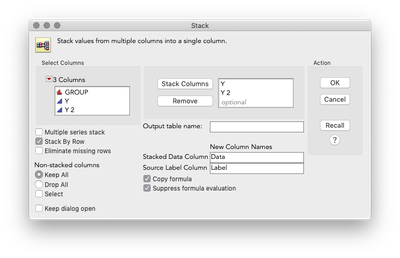``````Data Table( "Test" ) << Stack(
columns( :Y, :Y 2 ),
Source Label Column( "Label" ),
Stacked Data Column( "Data" )
);``````

This will return a table like below: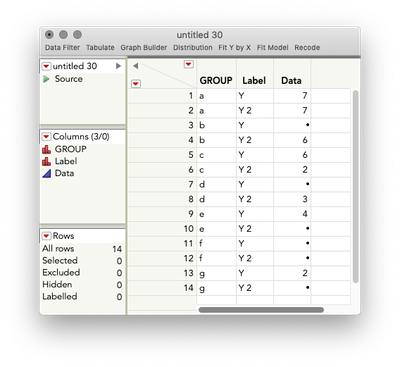Remove the missing values by right-clicking any of the cells that are missing > select matching. Then, delete those rows. This returns the attached table. Now, make your graph but use a local data filter rather than a column switcher to select what previously was a response from your Label Column:

``````Graph Builder(
Show Control Panel( 0 ),
Variables( X( :GROUP ), Y( :Data ) ),
Elements( Bar( X, Y, Legend( 4 ) ) ),
Local Data Filter(
columns( :Label ),
Where( :Label == "Y" ),
Display( :Label, Size( 160, 17 ) )
)
)
);``````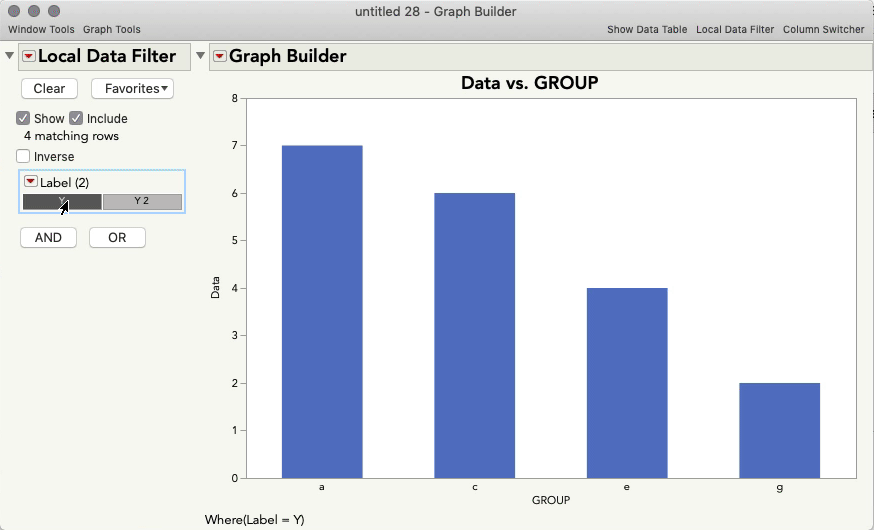Since there are no missing rows, Graph Builder displays only those levels of Group that are populated with data.  You can, of course, generalize this to any number of levels of Y -- simply stack all of them in the initial step.

I hope this helps!

Highlighted

## Re: Graph Builder: hide X categories with no data

Thanks @julian  for this suggestion! Indeed it allows to display only levels with data.

The only problem (but maybe I ask too much)  is that we lose the link between rows. For instance if I select the level "a" for Y and if I want to see the corresponding value for Y2, nothing is highlighted when I select Y2 with the local data filter.

Maybe coding the expected behavior is the solution...

HighlightedjulianCommunity Manager

## Re: Graph Builder: hide X categories with no data

Hi @anne_sa,

I see just what you mean. It really might come down to coding up something custom, but let me take one more interactive stab at this. Are there always only four levels (albeit different levels) of Group for each Y? If so, here's a potential hack: Graph Builder will maintain the X axis upon column switching, and since you have your levels ordered ascending (thus blank levels are always at the end), we could adjust the axis so that only four levels are showing. You can do this in the axis settings (right click the axis > axis settings), or just by dragging out the end of the axis until the missing levels are hidden).

``````Graph Builder(
Show Control Panel( 0 ),
Variables( X( :GROUP, Order By( :Y, Ascending, Order Statistic( "Mean" ) ) ), Y( :Y ) ),
Elements( Bar( X, Y, Legend( 4 ) ) ),
Column Switcher( :Y, {:Y, :Y 2} ),
SendToReport( Dispatch( {}, "GROUP", ScaleBox, {Min( -0.5 ), Max( 3.5 ), Inc( 1 ), Minor Ticks( 0 )} ) )
);``````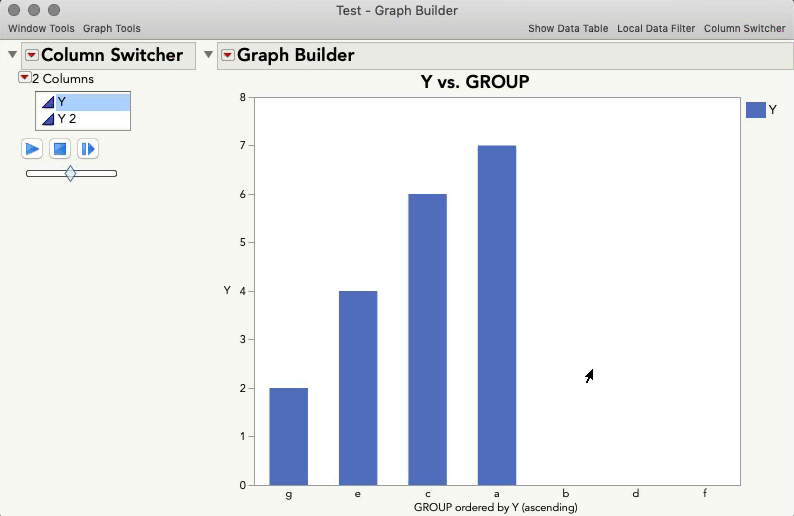Maybe a potential solution in your case?

Highlighted

## Re: Graph Builder: hide X categories with no data

Hi @julian!

I really appreciate your efforts to solve my problem! Unfortunately, the number of non-missing levels can be different from one response variable to the next one. However, your last post can definitely be used for the “coding solution”. Changing the axis settings based on the level number is indeed a nice way to display what is expected. I will work in that direction. Thanks for your help!

Highlighted

## Re: Graph Builder: hide X categories with no data

Hi Anne,

I wanted to check back with you to see if you have found a coding solution to your problem.  It took me a while to find the time but I have a coding solution if you still need one.

Highlighted

## Re: Graph Builder: hide X categories with no data

I have written something to basically update the column switcher and change the axis setting (as suggested by @julian ) each time we select a new variable. However it may not be very efficient so of course I am very interested by your solution!

Article Labels

There are no labels assigned to this post.